https://www.proz.com/kudoz/french-to-english/mathematics-statistics/6056029-papier-graphique-gausso-arithm%C3%A9tique.html

# papier graphique gausso-arithmétique

## English translation: normal probability (graph) paper / Gaussian probability graph paper / arithmetic probability paper

GLOSSARY ENTRY (DERIVED FROM QUESTION BELOW)
 French term or phrase: papier graphique gausso-arithmétique English translation: normal probability (graph) paper / Gaussian probability graph paper / arithmetic probability paper Entered by:

 20:35 Mar 1, 2016
French to English translations [PRO]
Tech/Engineering - Mathematics & Statistics / Statistics for the industry
 French term or phrase: papier graphique gausso-arithmétique "Pour étudier la normalité on aurait également pu utiliser le test de la droite de Henry. Cette méthode consiste en l’utilisation d’un papier graphique gausso-arithmétique, spécialement gradué sur lequel une courbe normale devient une ligne droite." I am looking for a specific kind of paper, which might be a kind of logarithmic paper but with some differencies concerning the vertical scale. You can find a pretty clear definition of it on the french wikipedia: https://fr.wikipedia.org/wiki/Droite_de_Henry and some images on google: https://www.google.fr/search?q=papier graphique gausso arith... thanks for your help !
 Marina DenogentFranceLocal time: 21:13
 probability graph paper / Gaussian probability graph paper / arithmetic probability paper Explanation:Found quite a few hits on Google, which would be worth your while looking at for any other posisbilities. This one seemd pretty convincing: A Random Walk Through Fractal Dimensions https://books.google.fr/books?isbn=3527615989 Brian H. Kaye - 2008 - ‎Technology & Engineering This type of graph paper is variously known as probability graph paper, Gaussian probability graph paper or arithmetic probability paper. Engineers, with their ...--------------------------------------------------Note added at 21 minutes (2016-03-01 20:57:09 GMT)--------------------------------------------------It has a symmetrically-stretched Y-axis scale on the left, corresponding to the shape of a Gaussian 'normal' distribution, with a +/– arithmetic scale on the right, enabling certain statistical factors to be read directly off the curve as plotted.
Selected response from:

Tony M
France
Local time: 21:13
 Thank you !4 KudoZ points were awarded for this answer

4 +3probability graph paper / Gaussian probability graph paper / arithmetic probability paper
 Tony M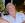Summary of reference entries provided
normal probability paper
 Roy vd Heijden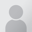19 mins   confidence:peer agreement (net): +3
probability graph paper / Gaussian probability graph paper / arithmetic probability paper

Explanation:
Found quite a few hits on Google, which would be worth your while looking at for any other posisbilities. This one seemd pretty convincing:

A Random Walk Through Fractal Dimensions

Brian H. Kaye - 2008 - ‎Technology & Engineering

This type of graph paper is variously known as probability graph paper, Gaussian probability graph paper or arithmetic probability paper. Engineers, with their ...

--------------------------------------------------
Note added at 21 minutes (2016-03-01 20:57:09 GMT)
--------------------------------------------------

It has a symmetrically-stretched Y-axis scale on the left, corresponding to the shape of a Gaussian 'normal' distribution, with a +/– arithmetic scale on the right, enabling certain statistical factors to be read directly off the curve as plotted.

 Tony MFranceLocal time: 21:13Native speaker of: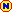EnglishPRO pts in category: 35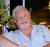Thank you !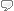Peer comments on this answer (and responses from the answerer)
agree
 58 mins
-> Thanks, Phil!

agree
 2 hrs
-> Merci, Daryo !

agree  claude-andrew: Yes. Got some by my left elbow!
 12 hrs

 1 hr peer agreement (net): +1
Reference: normal probability paper

Reference information:
« Le Normal Probability Plot, aussi appelé droite de Henry, est une analyse graphique pour vérifier si une série de données est répartie d’une manière « Normale ». Le principe est plutôt simple : à partir de nos données originelles, on va construire une série de données « théoriques » via la loi normale. En traçant un graphique représentant en X nos valeurs originelles et en Y nos valeurs théoriques, nous devrions obtenir une ligne droite si celles-ci sont normales. »
(http://www.wikilean.com/Articles/Analyse/9-Les-tests-d-hypot...

“In a normal probability plot (also called a "normal plot"), the sorted data are plotted vs. values selected to make the resulting image look close to a straight line if the data are approximately normally distributed. Deviations from a straight line suggest departures from normality. The plotting can be manually performed by using a special graph paper, called normal probability paper.”
(https://en.wikipedia.org/wiki/Normal_probability_plot)

“Normal probability paper is another graph paper with rectangles of variable widths. It is designed so that "the graph of the normal distribution function is represented on it by a straight line", i.e. it can be used for a normal probability plot.”
(https://en.wikipedia.org/wiki/Graph_paper)

--------------------------------------------------
Note added at 1 uur (2016-03-01 21:56:45 GMT)
--------------------------------------------------

"ARITHMETIC PROBABILITY PAPER Arithmetic Probability Paper—A graph paper, also called normal probability paper, with uniform subdivisions for one scale and the other scale ruled in such a way that a cumulative normal distribution plots as a straight line."

« Droite de Henri

C’est une pratique tombée en désuétude qui se réalisait sur un papier spécial dit papier gausso-arithmétique qui par anamorphose transformait la sigmoïde de la fonction de répartition de loi normale en une droite. D’après Funkhouser , l’intérêt d’une tel papier fut soulignée par Francis Galton en 1899  et introduit aux États-Unis d’Amérique en 1914 par Hazen  sous le nom de arithmetic probability paper. Un papier similaire fut introduit indépendamment en Angleterre par Dufton en 1930 sous le nom de permille paper , ce même auteur fit d’ailleurs remarquer  qu’il y avait une erreur dans l’article de Hazen . En France, la tradition remonte encore plus loin , le terme de droite de
Henri fait référence aux cours donnés en 1894 par le colonel Henri `a l’école d’artillerie Fontainebleau . Il a introduit un papier gausso-arithémtique pour vérifier la normalité de la portée de tir des canons (voir aussi  pour la solution de Lhoste à l’épineux problème de l’auto-corrélation temporelle des conditions de tir). Le terme de ”droite de Henry” ou ”droite de Henri”1 perdure en France.
1 l’orthographe des noms de famille n’a pas toujours été stable... »

--------------------------------------------------
Note added at 1 uur (2016-03-01 21:58:04 GMT)
--------------------------------------------------

 Roy vd HeijdenBelgiumNative speaker of:DutchPeer comments on this reference comment (and responses from the reference poster)
agree  DLyons: That's the usual name.
 42 mins